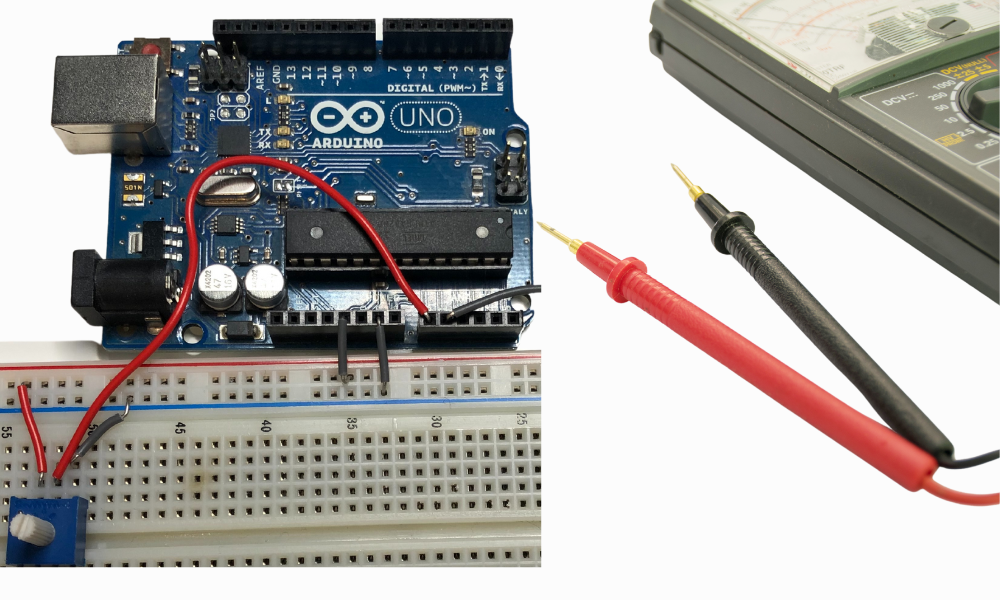Search

Updated: Apr 23, 2021

If you need to control knobs and potentiometers in your Arduino project and want them to affect things in a Processing sketch, you are going to need to read and use analog input.Here is a video demo of the project:

What you will learn
• Wiring potentiometers on a breadboard and into an Arduino

• Sending the analog values to a Processing sketch via Serial line

• Drawing a dynamic chart in Processing based of Serial input

Hardware

Arduino Wiring DiagramClose-Up of the ArduinoSoftware

Arduino Code

int sensorValueLeft = 0;     // value for left pot
int sensorValueRight = 0;    // value for right pot

void setup() {
// initialize serial communications
Serial.begin(9600);
}

void loop() {
// read the analog values from potentiometers

// print results to serial monitor
Serial.print(sensorValueLeft);
Serial.print(":");
Serial.print(sensorValueRight);
Serial.println();
delay(2);
}

Things to note:

• Setup serial communication. See Arduino Communication With Processing post for more detail on this setup.

• sensorValueLeft = analogRead(analogInPinLeft); - This is the basic syntax for obtaining an analog reading from a specific pin on the Arduino.

If we wire up the Breadboard, connect it to our machine, and then run the sketch and look at the Serial Monitor, we can see that the values for each Potentiometer are shown in the format sensorValueLeft:sensorValueRight. The analog values can range from 0 to 1023. This means that in the image below, the left potentiometer at 300 is turned only about 1/3 of its full range, and the right potentiometer at 1023 is turned all the way to the right as far as it can go.Here is the Processing sketch:

import processing.serial.*;

Serial myPort;
String data="";
int horizontal, vertical;

void setup() {
size (600, 600);
String portName = Serial.list();
myPort = new Serial(this, portName, 9600);
myPort.bufferUntil('\n');
background(128, 128, 128);
}

void draw() {

background(128, 128, 128);

// Title Text
textSize(45);
textAlign(CENTER);
fill(0, 0, 0);
text("Analog Values Demo", width / 2.0, 80);

// Left Rectangle
fill(80,32,240);
stroke(202,18,18);
rectMode(CENTER);
rect(width / 3.5, (height  - ((horizontal * 0.40) / 2) - 75), width / 4, horizontal * 0.40, 20);
textSize(45);
textAlign(CENTER);
fill(0, 0, 0);
text(String.valueOf(horizontal), width / 3.5, height - 10);

// Right Rectangle
fill(0,255,0);
stroke(0,18,18);
rectMode(CENTER);
rect(width / 1.5, (height - ((vertical * 0.40) / 2) - 75) , width / 4,vertical * 0.40, 20);
textSize(45);
textAlign(CENTER);
fill(0, 0, 0);
text(String.valueOf(vertical), width / 1.5, height - 10);

}

void serialEvent (Serial myPort) {
// reads the data from the Serial Port
// if you got any bytes other than the linefeed:
if (data != null) {
data = trim(data);
// split the string at ":"
String items[] = split(data, ':');
if (items.length > 1) {
//--- Roll,Pitch in degrees
horizontal = int(items);
vertical = int(items);
}
}
}

Things to note:

rect(width / 3.5, (height  - ((horizontal * 0.40) / 2) - 75), width / 4, horizontal * 0.40, 20);

These rect statements are where we use the analog values received from the Arduino via serial communication, and then render the rectangle with each frame. You can read the Processing Rect Documentation, where we are using this form of the constructor:

In our use case, we have:

xPosition = width / 3.5

Sets x coordinate to 1/3.5 the width of the sketch area.

yPosition = (height - ((horizontal * 0.40) / 2) - 75)

Sets y coordinate to 40% of the analog value for the left potentiometer, as measured from the bottom of the sketch + 75 units to account for the label. The value must also be divided by 2 because the coordinate specifies the center of the rectangle.

width = width / 4

Sets width to 1/4 the width of the sketch area.

height = horizontal * 0.40

Sets height coordinate to 40% of the analog value for the left potentiometer.